# 1 Thursday, August 19

## 1.1 Intro and Overview

Course website: https://akramalishahi.github.io/CharClass.html

Description from Akram’s syllabus:

This course is about characteristic classes, which are cohomology classes naturally associated to vector bundles or, more generally, principal bundles. They are a key tool in modern {algebraic, differential}×{topology, geometry}. The course starts with an introduction to vector bundles and principal bundles. It then discusses their main characteristic classes—the Euler class, Stiefel-Whitney classes, Chern classes, and Pontryagin classes. The last part of the class discusses some applications of characteristic classes to bordisms. In the process, we will see some nice applications (e.g., to immersions) and review some important parts of algebraic topology (e.g., obstruction theory).

References:

• [Hu?] Husemoller, Fiber bundles.
• [MS?] Milnor and Stasheff, Characteristic classes.
• [S?] Steenrod, The topology of fibre bundles.
• [Ha?] Hatcher, Vector bundles and K-theory .
• [BottTu?] Bott and Tu, Differential forms in algebraic topology.

Prerequisites:

• Smooth manifolds: smooth maps and derivatives, differential forms.
• Algebraic topology: homology and cohomology.

An overview of what we’ll cover:

• General definitions and constructions related to vector bundles and fiber bundles.

Why bundles? For a bundle $$E \xrightarrow{\pi} B$$, characteristic classes will be cohomology classes in $$H^*(B)$$. Natural examples include

• The tangent bundle $$TX\to X$$, and vector fields will be sections.
• Exterior products $$\bigwedge\nolimits^n TX$$, where differential forms live
• Normal bundles $$\nu$$, giving directions an embedded submanifold can be deformed.

Also note that manifolds locally look like vectors spaces ($${\mathbb{R}}^n$$!) and so embedded manifolds locally look like vector bundles. In particular, if $$f: M^n \hookrightarrow N^k$$ is an embedding, locally $$\nu$$ is locally a $$k-n$$ dimensional vector bundle over $${\mathbb{R}}^n$$ (and globally a bundle of the form $$\nu: E \to f(M_n)$$)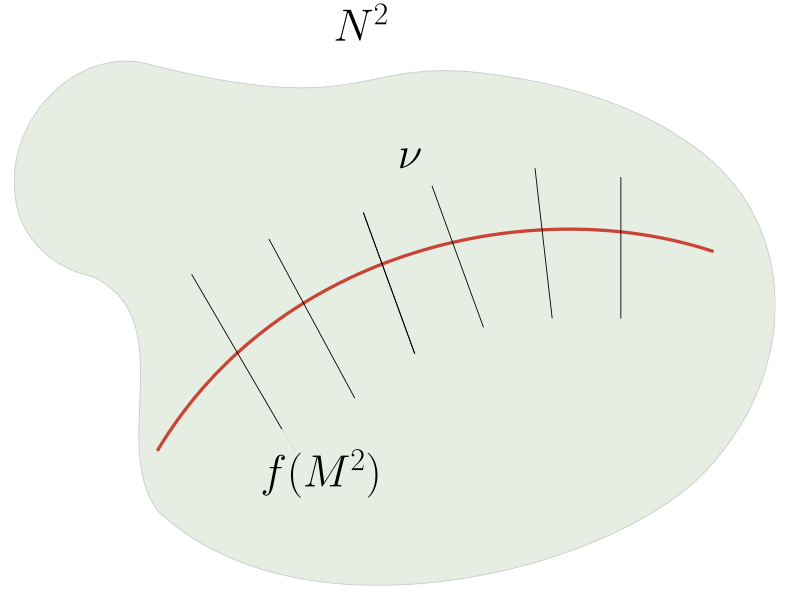• Characteristic Classes: Euler, Stiefel-Whitney, Pontryagin, etc.

These package geometric information into algebraic invariants that are often computable. Some examples:

• Stiefel-Whitney classes can detect if $$M^n = {{\partial}}M^{n+1}$$ is a boundary (for smooth closed manifolds).
• Euler classes can prove the Hairy Ball theorem, i.e. $$S^2$$ admits no nonvanishing continuous vector fields, which can be generalized to $$S^{2n}$$ and to splitting the tangent bundle.
• Pontryagin classes: Milnor used these to produce exotic $$S^7$$s! These are manifolds $$M^7$$ which are homeomorphic but not diffeomorphic to $$S^7$$.
• Chern classes.

## 1.2 Fiber Bundles

A fiber bundle over $$B$$ with fiber $$F$$ is a continuous map $$\pi: E\to B$$ where each $$b\in B$$ admits an open neighborhood $$U \subseteq B$$ and a homeomorphism $$\phi: \pi^{-1}(U)\to U\times F$$ such that the following diagram commutes in $${\mathsf{Top}}$$:Here the square is $$[0, 1]^{\times 2}$$.

Note that this necessarily implies that all fibers are homeomorphic, noting that $$F_b \mathrel{\vcenter{:}}=\pi^{-1}(b) \xrightarrow{\phi} \left\{{b}\right\} \times F$$. We have inclusions: vector bundles $$\implies$$ fiber bundles $$\implies$$ fibrations. For a fibration that’s not a fiber bundle, one can collapse a fiber in a trivial bundle, e.g.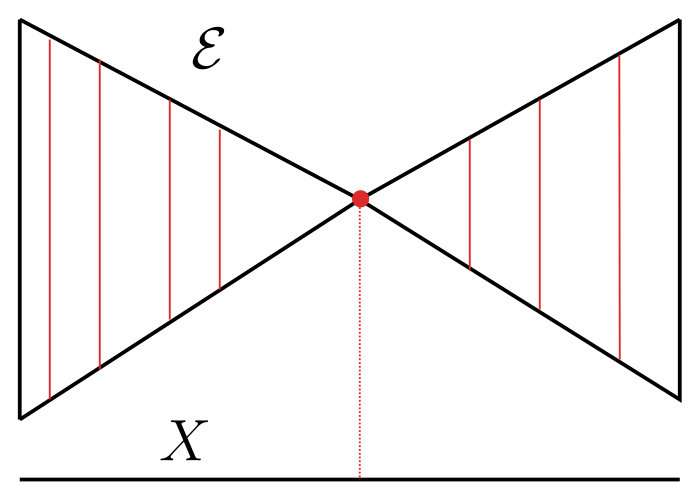An atlas bundle for $$\pi:E\to B$$ is a collection of charts $$\left\{{(U_\alpha, \phi_\alpha)}\right\}_{\alpha\in I}$$ such that $$\left\{{U_\alpha}\right\}\rightrightarrows B$$.

• $$E \mathrel{\vcenter{:}}= B\times F \xrightarrow{p_2} F$$ the trivial/product bundle.

• $$\widehat{X} \to X$$ any covering space. Note that the fibers are discrete.

• The Möbius band:

<– Xournal file: /home/zack/SparkleShare/github.com/Notes/Class_Notes/2021/Fall/CharacteristicClasses/sections/figures/2021-08-19_13-41.xoj –>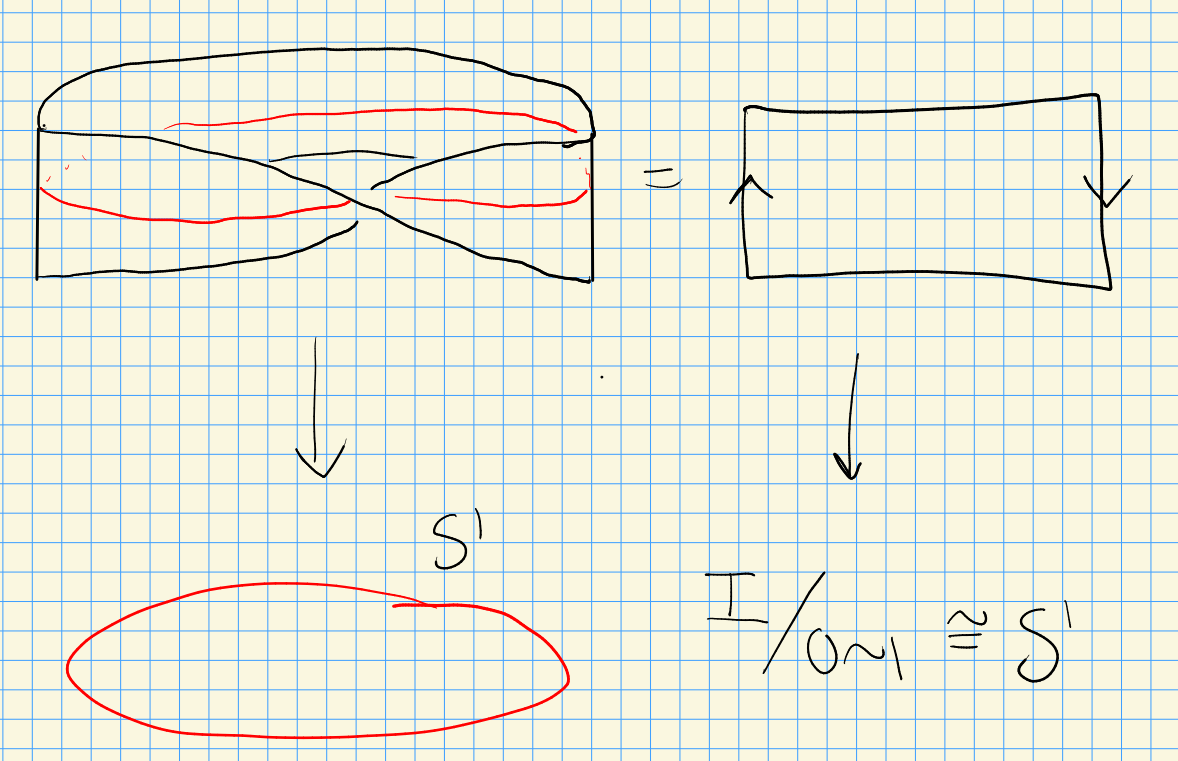This is a fiber bundle with fibers $$[0, 1]$$. For a fiber bundle, include the boundary, but to make this a vector bundle do not include it!

Consider the following setup:

• $$B\in {\mathsf{Top}}$$
• $$\pi:E\to B$$ is a map of underlying sets
• There is a bundle atlas $$\left\{{\phi_\alpha}\right\}$$, each $$\phi_\alpha$$ being a bijection.

Then there exists at most one topology on $$E$$ such that $$\pi: E\to B$$ is a fiber bundle with the given atlas.

Find necessary conditions for at least one topology to exist!

## 1.3 Vector Bundles

An $$n{\hbox{-}}$$dimensional real (resp. complex) vector bundle over $$B$$ is a fiber bundle $$\pi:E\to B$$ along with a real vector space structure on each fiber $$F_b$$ such that for each $$b\in B$$ there exists a neighborhood $$U \ni b$$ and a chart $$(U, \phi: \pi^{-1}(U) \to U\times {\mathbb{R}}^n)$$ (resp. $${\mathbb{C}}^n$$) where $${ \left.{{\phi}} \right|_{{F_b}} }: F_b \xrightarrow{\sim} {\mathbb{R}}^n$$ (resp. $${\mathbb{C}}^n$$) is an isomorphism of vector spaces.

• The trivial (product) bundle $$B\times{\mathbb{R}}^n \xrightarrow{p_1} B$$.
• The tangent bundle $$T X$$.
• Identifying the Möbius band as $$[0, 1] \times(0, 1)/\sim$$ as $$I \times{\mathbb{R}}/ (0, t)\sim (1, -t)$$ yields a 1-dimensional bundle $$M\to S^1$$.

We have some natural operations:

1. Direct sums.

For $$E_1, E_2 \in { { {\mathsf{Bun}}\qty{\operatorname{GL}_r} }}_{/ {B}}$$, so $$E_1 \xrightarrow{\pi_1} B$$ and $$E_2 \xrightarrow{\pi_2} B$$, we can form $$E_1 \oplus E_2 \xrightarrow{\pi} B$$. As a set, take \begin{align*} E_1 \oplus E_2 \mathrel{\vcenter{:}}=\displaystyle\bigcup_{b\in B} F_{1, b} \oplus F_{2, b} \end{align*} as a union of direct sums of vector spaces. For the bundle map, take $$\pi(F_{1, b} \oplus F_{2, b}) \mathrel{\vcenter{:}}=\left\{{b}\right\}$$. For charts, for any $$b\in B$$ pick individual charts about $$b$$, say $$(U_1, \phi_1)$$ for $$E_1$$ and $$(U_1, \phi_2)$$ for $$E_2$$, form charts \begin{align*} \left\{{ (U_1 \cap U_2, \phi: \pi^{-1}(U_1 \cap U_2) \to {\mathbb{R}}^{n_1 + n_2})}\right\} \end{align*} where $$n_1 \mathrel{\vcenter{:}}=\dim_{\mathbb{R}}F_{1, b}$$ and $$n_2 \mathrel{\vcenter{:}}=\dim_{\mathbb{R}}F_{2, b}$$ and define $$(b, (v_1, v_2)) \xrightarrow{\phi} (\phi_1(v_1), \phi_2(v_2))$$.

# 2 Fiber Bundles with Structure and Principal $$G{\hbox{-}}$$ Bundles (Tuesday, August 24)

Setup:

• $$B \in {\mathsf{Top}}$$ is a space.
• $$\pi:E\to B$$ is a map of sets with fibers/preimages $$F \mathrel{\vcenter{:}}= F_b \mathrel{\vcenter{:}}=\pi^{-1}(\left\{{b}\right\})$$.
• A bundle atlas for $$\pi$$ is $$\phi$$ where $$\phi_U: \pi^{-1}(U) \to U \times F$$ is a bijection of sets

Then there is at most one topology on $$E$$ making $$\pi:E\to B$$ into a fiber bundle with the specified atlas.

Given a vector bundle $$\pi:E\to B$$, form the dual bundle $$\pi {}^{ \vee }:E {}^{ \vee }\to B$$ by setting

• $$E {}^{ \vee }\mathrel{\vcenter{:}}={\textstyle\coprod}_{b\in B} F_b {}^{ \vee }$$
• Set $$\pi {}^{ \vee }(F_b {}^{ \vee }) = \left\{{b}\right\} \in B$$.
• Given $$\phi: \pi^{-1}(U)\to U\times {\mathbb{R}}^n$$, set \begin{align*} \phi {}^{ \vee }: (\pi {}^{ \vee })^{-1}(U) = {\textstyle\coprod}_{b\in U} F_b {}^{ \vee }\longrightarrow U\times({\mathbb{R}}^n) {}^{ \vee }\cong U\times{\mathbb{R}}^n .\end{align*}

Here $$A \subseteq \pi^{-1}(U)$$ is open iff $$\phi_U(A)$$ is open in $$B$$.

Consider what happens on overlapping charts – looking at maps fiberwise yields:Starting at $$(U \cap V)\times F$$ and running the diagram clockwise yields a map \begin{align*} \phi_V \circ \phi_U ^{-1}: (U \cap V ) \times F &\to (U \cap V ) \times F \\ (b, f) &\mapsto (b, \phi_{VU}(f) ) \end{align*} where $$\phi_{VU}$$ is the following continuous map, defining a transition function: \begin{align*} \phi_{VU}: U \cap V &\to {\operatorname{Homeo}}(F) ,\end{align*} where $${\operatorname{Homeo}}(F) \mathrel{\vcenter{:}}=\mathop{\mathrm{Hom}}_{{\mathsf{Top}}}(F, F)$$ with the compact-open topology.

Let $$\mathop{\mathrm{Maps}}(X, Y)\mathrel{\vcenter{:}}=\mathop{\mathrm{Hom}}_{\mathsf{Top}}(X, Y)$$ be the set of continuous maps $$X\to Y$$, then a map $$Z\to \mathop{\mathrm{Maps}}(X, Y)$$ is continuous iff the following map is continuous: \begin{align*} Z\times X &\to Y \\ (z, x) &\mapsto f(z)(x) .\end{align*}

If $$X$$ is Hausdorff and locally compact then $$\mathop{\mathrm{Maps}}(X, Y)$$ will have this property for all $$Y$$. A subbasis for this topology will be given by taking $$C \subseteq X$$ compact, $$U \subseteq Y$$ open and taking the basic opens to be \begin{align*} S(C, U) \mathrel{\vcenter{:}}=\left\{{f\in \mathop{\mathrm{Maps}}(X, Y){~\mathrel{\Big\vert}~}f(X) \subseteq U}\right\} .\end{align*} If $$Y$$ has a metric, then this will coincide with the compact convergence topology, which has a basis \begin{align*} \left\{{ S(f, C, E) {~\mathrel{\Big\vert}~}C \subseteq X \text{ compact}, \forall {\varepsilon}>0,\,\forall f\in \mathop{\mathrm{Maps}}(X, Y) }\right\}, \\ S(f, C, E) \mathrel{\vcenter{:}}=\left\{{ g\in \mathop{\mathrm{Maps}}(X, Y) {~\mathrel{\Big\vert}~}d( f(x), g(x) ) < {\varepsilon}\,\,\forall x\in C }\right\} .\end{align*}

Let $$G \subseteq {\operatorname{Homeo}}(F)$$, then a fiber bundle with structure group $$G$$ is a fiber bundle $$F\to E \xrightarrow{\pi} B$$ together with a bundle atlas such that $$G \subseteq {\operatorname{Homeo}}(F)$$.

An $${\mathbb{R}}^n{\hbox{-}}$$bundle is just a bundle where $$F = {\mathbb{R}}^n$$ for all fibers, where we ignore the vector space structure and only take transition functions to be homeomorphisms. An $${\mathbb{R}}^n{\hbox{-}}$$bundle with a $$G\mathrel{\vcenter{:}}=\operatorname{GL}_n({\mathbb{R}})$$ is exactly a vector bundle, where we can use the structure group to put a vector space structure on the fibers. We have charts $$\phi_U: \pi^{-1}(U)\to U \times{\mathbb{R}}^n$$, so for all $$b\in U$$, writing $$F_b \mathrel{\vcenter{:}}=\pi^{-1}(\left\{{b}\right\})$$ and get $$\phi_U(F_b) = {~\mathrel{\Big\vert}~}{b} \times{\mathbb{R}}^n$$. We can then define addition and multiplication for $$w_1, w_2 \in F_b$$ as \begin{align*} cw_1+ w_2 \mathrel{\vcenter{:}}=\phi_U^{-1}\qty{ c\phi_U(w_1) + \phi_U(w_2) } .\end{align*} This is well-defined because for any other chart containing $$V\ni b$$, we have $$\phi_{VU}\in \operatorname{GL}_n({\mathbb{R}})$$. This follows by just setting $$A \mathrel{\vcenter{:}}=\phi_V \circ \phi_U ^{-1}$$ and writing \begin{align*} \phi_V(w_1 + w_2) &= A \phi_U(w_1 + w_2) \\ &\mathrel{\vcenter{:}}= A\qty{\phi_U(w_1) + \phi_U(w_2) } \\ &= A\phi_U(w_1) + A\phi_U(w_2) \\ &= \phi_V(w_1) + \phi_V(w_2) \\ &\mathrel{\vcenter{:}}=\phi_V(w_1 + w_2) .\end{align*}

An $${\mathbb{R}}^n{\hbox{-}}$$bundle with a $$\operatorname{GL}_{n}^+({\mathbb{R}})$$ structure is an orientable vector bundle, where \begin{align*} \operatorname{GL}_n^+({\mathbb{R}}) = \left\{{ A \in \operatorname{GL}_n({\mathbb{R}}) {~\mathrel{\Big\vert}~}\operatorname{det}(A) > 0 }\right\} .\end{align*}

A $$G\mathrel{\vcenter{:}}= O_n({\mathbb{R}})$$ structure yields vector bundles with Riemannian metrics on fibers, where $$O_n({\mathbb{R}}) \mathrel{\vcenter{:}}=\left\{{ A\in \operatorname{GL}_n({\mathbb{R}}) {~\mathrel{\Big\vert}~}AA^t = \operatorname{id}}\right\}$$. Here we use the fact that there is an equivalence between metrics (symmetric bilinear pairings) and choices of an orthonormal basis, e.g. using that if $$\left\{{e_1, \cdots, e_n}\right\}$$, one can specify an inner product completely by writing \begin{align*} v\mathrel{\vcenter{:}}=\sum v_i e_i,\quad w \mathrel{\vcenter{:}}=\sum w_i e_i &&\implies {\left\langle {v},~{w} \right\rangle} = \sum v_i w_i .\end{align*}

A principal $$G{\hbox{-}}$$bundle is a fiber bundle $$\pi:P\to B$$ with a right $$G{\hbox{-}}$$action $$\psi: P\times G\to P$$ such that

1. $$\psi\qty{F_b} = F_b$$, so the action preserves each fiber, and
2. $$\psi$$ is free and transitive.

# 3 Principal $$G{\hbox{-}}$$bundles (Thursday, August 26)

Today: relating $$\mathop{\mathrm{Prin}}{\mathsf{Bun}}_{/ {G}}$$ to fiber bundles with a $$G{\hbox{-}}$$structure. Recall that a principal $$G{\hbox{-}}$$bundle is a fiber bundle $$\pi:P\to B$$ with a fiberwise $$G{\hbox{-}}$$action $$P\times G\to P$$ which induces a free and transitive action on each fiber. Note that we assume $$G\in{\mathsf{Top}}{\mathsf{Grp}}$$. Any bundle in $$\mathop{\mathrm{Prin}}{\mathsf{Bun}}_{/ {G}}$$ is a fiber bundle with fibers $$F$$ homeomorphic to $$G$$ and admits a $$G{\hbox{-}}$$structure: \begin{align*} G &\hookrightarrow{\operatorname{Homeo}}(G) \\ g &\mapsto (h\mapsto gh) .\end{align*} Using that $$F\cong G$$, taking charts $$(U, \varphi), (V, \psi)$$ for $$\pi:P\to B$$, we can identifySo every transition function is given by left-multiplication by some element in $$G$$, as opposed to arbitrary homeomorphisms of $$G$$ as a topological group.

• Trivial actions: $$B \times G \xrightarrow{p_1} B$$.

• Regular covering spaces $$\pi:\tilde X\to X$$, then $$G = \mathop{\mathrm{Deck}}(\tilde X/X)$$ with the discrete topology.

• Given an $$n{\hbox{-}}$$dimensional vector bundle $$\pi: E\to B$$, take \begin{align*} \mathop{\mathrm{Frame}}(F_b) \mathrel{\vcenter{:}}=\left\{{(e_1, \cdots, e_n) \in F_b}\right\} \subseteq F_b^{\times n} ,\end{align*} the collection of all ordered bases of $$F_b$$. Then set \begin{align*} \mathop{\mathrm{Frame}}_n \mathrel{\vcenter{:}}={\textstyle\coprod}_{b\in B} \mathop{\mathrm{Frame}}(F_b) \to B \end{align*} to get a principal $$G{\hbox{-}}$$bundle for $$G = \operatorname{GL}_n(F_b)$$ under the following action: picking a framing $$(e_1, \cdots, e_n)$$ in $$F_b$$, then for $$A\in \operatorname{GL}_n(F_b)$$ regarded as a linear map, define \begin{align*} (\mathbf{e}_1, \cdots, \mathbf{e}_n) \cdot A \mathrel{\vcenter{:}}=\qty{\sum_i a_{i,1} \mathbf{e}_i, \sum_i a_{i, 2} \mathbf{e}_i, \cdots, \sum_i a_{i, n} \mathbf{e}_i } .\end{align*}

• Given an oriented $$n{\hbox{-}}$$dimensional vector bundle $$\pi:E\to B$$, one gets a $$G\mathrel{\vcenter{:}}=\operatorname{GL}_n^+({\mathbb{F}}_b)$$ by taking positively oriented frames.

• Given a vector bundle with a Riemannian metric, we get a principal $${\mathcal{O}}_n({\mathbb{R}}){\hbox{-}}$$bundle by taking orthonormal frames.

Given two principal $$G{\hbox{-}}$$bundles $$\pi: P\to B$$ and $$\pi': Q\to B$$, an isomorphism of principal bundles is a $$G{\hbox{-}}$$equivariant map $$P \xrightarrow{f} Q$$ commuting over $$B$$:Here equivariant means commuting with the $$G{\hbox{-}}$$action, in the following precise sense: let $$(U, \varphi)$$ and $$(V, \psi)$$ be charts for $$\pi, \pi'$$, then consider the composition \begin{align*} F: \qty{ (U \cap V) \times F \xrightarrow{\phi^{-1}} \pi^{-1}(U \cap V) \xrightarrow{f} (\pi')^{-1}(U \cap V) \xrightarrow{\psi} (U \cap V) \times F} .\end{align*} Note that this fixes every point $$b\in U \cap V$$, so we can regard $$F: U \cap V \to {\operatorname{Homeo}}(F)$$, using that $$f$$ commutes with the projection maps: \begin{align*} (b, ?) \mapsto \pi^{-1}(b) \mapsto (f\circ \pi^{-1})(b) = (\pi')^{-1}b \mapsto b .\end{align*} We say $$f$$ is a $$G{\hbox{-}}$$isomorphism iff $$F$$ sends everything to $$G$$.

## 3.1 Sending Fiber Bundles to Principal $$G{\hbox{-}}$$bundles

Given a principal $$G{\hbox{-}}$$bundle $$\pi:P\to B$$ and a $$F\in {\mathsf{Top}}$$ with a left $$G{\hbox{-}}$$action. Then define \begin{align*} P { \underset{\scriptscriptstyle {G} }{\times} } F / (pg, f)\sim (p, gf) \end{align*} as a fiber bundle over $$B$$ using $$\pi$$ as the projection. Note that this looks like a tensor product, and this works in general for any space $$P$$ with a right $$G{\hbox{-}}$$action and $$F$$ with a left $$G{\hbox{-}}$$action. This will be a fiber bundle with fiber $$F$$ and structure group $$G \leq {\operatorname{Homeo}}(F)$$.

Locally there is a homeomorphism: \begin{align*} (U\times G) \overset{\scriptscriptstyle {G} }{\times} F &\xrightarrow{\sim} U\times F \\ (p, g, f) &\mapsto (p, gf) .\end{align*} This is well defined since $$(p, gh, f)$$ and $$(p, g, hf)$$ map to $$(p, ghf)$$. The inverse is $$(p, f) \mapsto (p, 1, gf)$$.

Check that this is a fiber bundle with $$G{\hbox{-}}$$structure.

# 4 Tuesday, August 31

We want to show the equivalence between (isomorphism classes) of fiber bundles with $$G$$ structures with fiber $$F$$ and principal $$G{\hbox{-}}$$bundles. Recall that $$\mathop{\mathrm{Prin}}{\mathsf{Bun}}_{/ {G}}$$ are fiber bundles $$P \xrightarrow{\pi} B$$ with a right fiberwise $$G{\hbox{-}}$$action which is free and transitive on each fiber.

To send fiber bundles to principal bundles, we used a mixing construction. Since $$G\curvearrowright F$$, we get an identification $$G \subseteq {\operatorname{Homeo}}(F, F)$$. We constructed \begin{align*} P { \underset{\scriptscriptstyle {G} }{\times} } F \mathrel{\vcenter{:}}=(P\times F)/(pg, f)\sim (p, gf) .\end{align*} A lemma was that $$P{ \underset{\scriptscriptstyle {G} }{\times} } F \to B$$ is a fiber bundle with fiber $$F$$ and projection $$\pi(p, f) \mathrel{\vcenter{:}}=\pi(p)$$.

Today we’ll talk about the reverse direction. Note the composition of sending $$E$$ to $$\mathop{\mathrm{Prin}}{\mathsf{Bun}}_{/ {G}}$$ and then mixing recovers $$E$$ when $$E$$ is a vector bundle, but not generally. \begin{align*} \left\{{\substack{ \text{Fiber bundles with }G{\hbox{-}}\text{structures} \\ \text{ and fiber }F }}\right\} \adjunction{\text{Clutching}}{\text{Mixing}}{}{} \left\{{\substack{ \text{Principal $G{\hbox{-}}$bundles} }}\right\} \end{align*}

For $$E \xrightarrow{\pi} B$$ a real vector bundle, we sent it to $$\mathop{\mathrm{Frame}}(E)$$, which is a principal $$\operatorname{GL}_n({\mathbb{R}}){\hbox{-}}$$action Using a left action $$\operatorname{GL}_n \curvearrowright{\mathbb{R}}^n$$, we can form $$\mathop{\mathrm{Frame}}(E) { \underset{\scriptscriptstyle {\operatorname{GL}_n} }{\times} } {\mathbb{R}}^n$$, a fiber bundle with a $$G \mathrel{\vcenter{:}}=\operatorname{GL}_n$$ structure, i.e. exactly a vector bundle.

Show that there is a homeomorphism \begin{align*} \mathop{\mathrm{Frame}}(E) { \underset{\scriptscriptstyle {\operatorname{GL}_n} }{\times} } {\mathbb{R}}^n \xrightarrow{\sim}E .\end{align*}

For the reverse map, take a map $$f$$ defined by $$(\mathbf{e}_1, \cdots, \mathbf{e}_n) \in \pi^{-1}(b) \subset \mathop{\mathrm{Frame}}(E)$$ and $${\left[ {{ {b}_1, {b}_2, \cdots, {b}_{n}}} \right]}^t \in {\mathbb{R}}^n$$ to $$\sum_{i=1}^n b_i \mathbf{e}_i$$. For this to be well-defined, one needs to show the following: \begin{align*} f(( \mathbf{e}_1, \cdots, \mathbf{e}_n) A, \mathbf{b}) = f( (\mathbf{e}_1, \cdots, \mathbf{e}_n), A\mathbf{b}) && \forall A\in \operatorname{GL}_n({\mathbb{R}}) .\end{align*} The left hand side is \begin{align*} b_1 (a_{1, 1} \mathbf{e}_1 + \cdots + a_{n, 1} \mathbf{e}_n ) + \cdots + b_n(a_{1, n} \mathbf{e}_1 + \cdots + a_{n, n} \mathbf{e}_n) = \sum_{i=1}^n b_i \qty{ \sum_{j=1}^n a_{j, i} \mathbf{e}_j } .\end{align*}

The right-hand side is \begin{align*} (a_{1, 1}b_1 + \cdots + a_{1, n}b_n)\mathbf{e}_1 + \cdots + (a_{n, 1} b_1 + \cdots + a_{n,n} b_n)\mathbf{e}_n = \sum_{i=1}^n \qty{\sum_{j=1}^n a_{i, j} b_i } \mathbf{e}_i ,\end{align*} and one can check that these sums match term by term.

Note that if we choose a basis for the fibers, we can set $$A' \mathrel{\vcenter{:}}={\left[ {\mathbf{e}_1, \cdots, \mathbf{e}_n} \right]}^t$$ to be the matrix with columns $$\mathbf{e}_i$$, the map $$f$$ is given by $$f(A', \mathbf{b}) \mathrel{\vcenter{:}}= A'\mathbf{b}$$, and we’re showing that $$(A'A)\mathbf{b} = A'(A\mathbf{b})$$. However, this involves choosing an isomorphism between the abstract fibers and $${\mathbb{R}}^n$$.

What are local charts for a principal bundle? For $$P{ \underset{\scriptscriptstyle {G} }{\times} } F$$, pick charts $$(U, \phi)$$ for $$P \xrightarrow{\pi} B$$: \begin{align*} \phi: \pi^{-1}(U) &\to U \times G \\ x & \mapsto (\pi(x), \gamma(x)) .\end{align*} Then a local chart for the principal bundle is of the form \begin{align*} \pi^{-1}(U) { \underset{\scriptscriptstyle {G} }{\times} } F &\xrightarrow{\tilde \phi} U \times F \\ (x, f) &\mapsto ( \pi(x), \gamma(x) f) .\end{align*} We also have \begin{align*} (U \times G){ \underset{\scriptscriptstyle {G} }{\times} } F &\to U \times F \\ ( (x, g), f) &\mapsto (x, gf) .\end{align*} One can invert $$\tilde \phi$$ using $$(a, f) \mapsto (\phi^{-1}(a, 1), f)$$. This yields transition functions: writing \begin{align*} \phi_V: \pi^{-1}(V) &\to V \times G \\ x &\mapsto (\pi(x), \psi(x) ) ,\end{align*} then \begin{align*} \phi_{VU} = \phi_V \circ \phi_U^{-1}: (a, f) & \\ &\xrightarrow{\phi_U^{-1}} ( \phi^{-1}_U(a, 1), \,\, f) \\ &\xrightarrow{\phi_V} (\pi \phi_U^{-1}(a, 1), \,\, \psi( \phi_U^{-1}(a, 1))f ) \\ &= (a,\,\, \psi(\phi_U^{-1}(a, 1)) f) .\end{align*} This says that $$(a, 1) \mapsto \psi( \phi^{-1}_U(a, 1))$$.

In general, for a bundle $$E \xrightarrow{\pi} B$$, taking local trivializations $$\phi_U, \phi_V$$, we get $$\phi_{VU}: (U \cap V ) \times F {\circlearrowleft}$$, or currying an argument, $$\phi_{VU}: U \cap V \to {\operatorname{Homeo}}(F, F)$$. If the bundle satisfies the cocycle condition $$\phi_{UW} = \phi_{VW} \circ \phi_{UV}$$. Given a covering $$\left\{{U_i}\right\}_{i\in I}\rightrightarrows B$$, we get $$\phi_{ij}: U_i \cap U_j \to G$$ and a topological space $$F$$ with $$G \subseteq {\operatorname{Homeo}}(F, F)$$ satisfying the cocycle condition $$\phi_{ik} = \phi_{jk} \circ \phi_{ij}$$, then we can build a fiber bundle with fiber $$F$$ and structure group $$G$$ by setting $$E = {\textstyle\coprod}_{i\in I} (U_i \times F)/\sim$$ We then set for $$b\in U_i \cap U_j$$ the equivalence \begin{align*} (U_i \times F) \ni (b, f)\sim (b, \phi_{ij}(b) f) \in (U_j \times F) .\end{align*} This is an equivalence relation precisely when the cocycle condition holds. This is referred to as clutching data.

Let $${\mathbb{Z}}/2\curvearrowright{\mathbb{R}}$$ by $$t\mapsto -t$$ with $$U, V$$ defined as follows: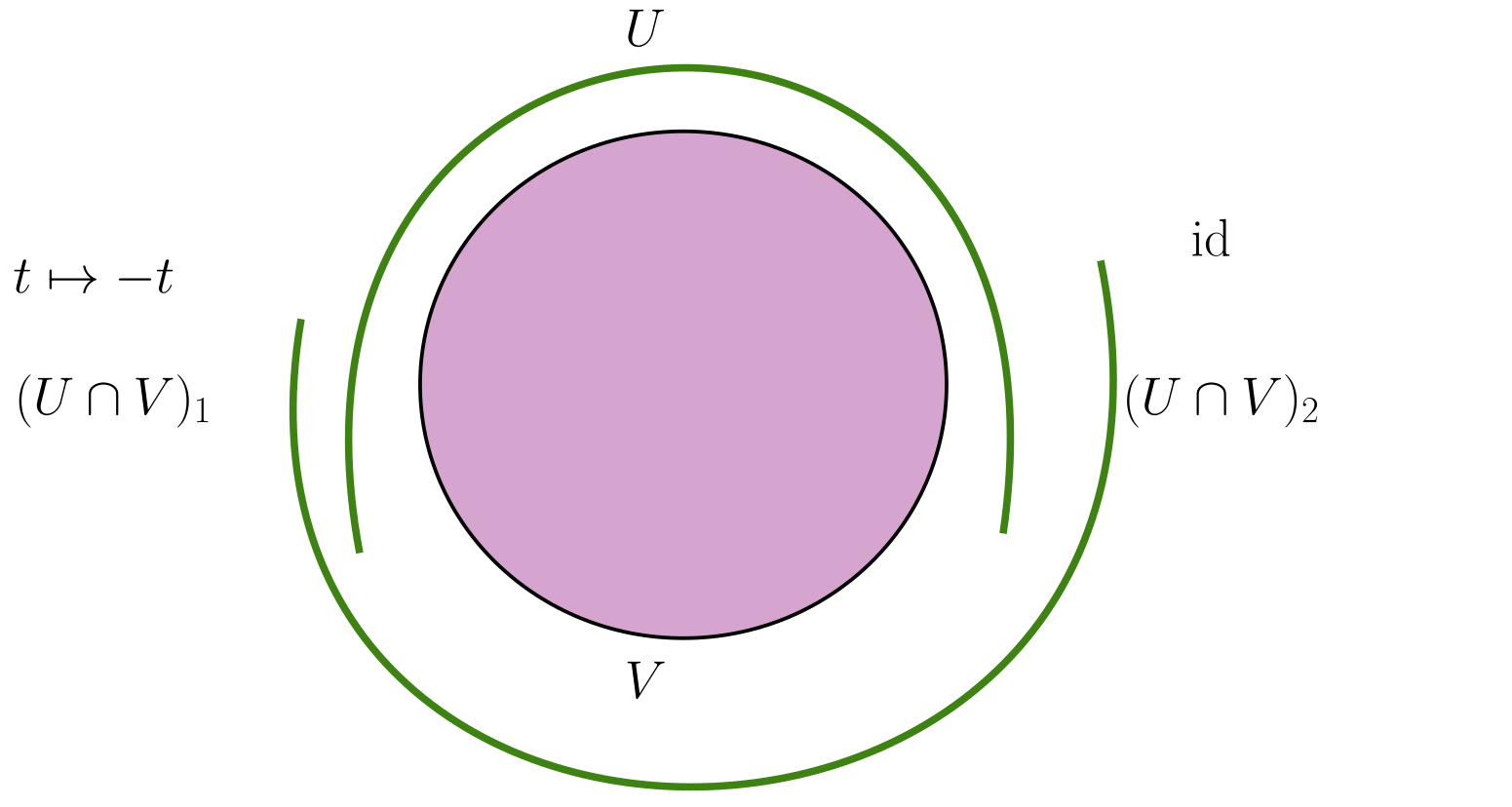Labeling the intersections as 1, 2, we set \begin{align*} \phi_{VU}: (U \cap V) = (U \cap V)_1 {\textstyle\coprod}(U \cap V)_2 &\to {\mathbb{Z}}/2 && \subseteq {\operatorname{Homeo}}({\mathbb{R}}) \\ x {\textstyle\coprod}y &\mapsto x {\textstyle\coprod}-y .\end{align*} This yields the open Mobius band.

Actually, several questions. Assume $$F$$ is a fixed fiber common to all of the following constructions, since bundles with non-homeomorphic fibers can’t be isomorphic.

1. Given clutching data $$\left\{{\phi_{ij}}\right\}$$, when is the resulting fiber bundle trivial?
2. Given two sets of clutching data $$\left\{{\phi_{ij}}\right\}$$ and $$\left\{{\psi_{ij}}\right\}$$ with the same open cover $$\left\{{U_i}\right\} \rightrightarrows X$$, when are the corresponding bundles $$G{\hbox{-}}$$isomorphic?
3. Given two sets of clutching data $$\left\{{\phi_{ij}}\right\}$$ and $$\left\{{\psi_{ij}}\right\}$$ with the different open cover $$\left\{{U_i}\right\} \rightrightarrows X$$ and $$\left\{{V_i}\right\} \rightrightarrows X$$, when are the corresponding bundles $$G{\hbox{-}}$$isomorphic?

The fiber bundle obtained from $$\phi_{ij}$$ is trivial iff there exists a map $$\gamma_i: U_i \to G$$ such that $$\phi_{ij} = \gamma_i \gamma_j^{-1}$$.

The trivial bundle is $$B\times F\to B$$, so if we have $$E\to B$$, we can take a map \begin{align*} U_i \times F &\to U_i \times F \\ (b, f) &\mapsto (b, \gamma_i(b) f) .\end{align*} Use that $$B\times F$$ is a trivial bundle, so it is its own trivialization.

To be continued next time.

# 5 Thursday, September 02

Recall that we have a correspondence \begin{align*} \left\{{\substack{ \text{Vector bundles }E }}\right\} &\adjunction{\text{clutching}}{\text{mixing}}{}{} \left\{{\substack{ \text{Principal $\operatorname{GL}_n{\hbox{-}}$bundles $\mathop{\mathrm{Frame}}(E)$} }}\right\} \end{align*} We saw that $$E \cong \mathop{\mathrm{Frame}}(E) { \underset{\scriptscriptstyle {\operatorname{GL}_n({\mathbb{R}})} }{\times} } {\mathbb{R}}^n$$. If we take $$\mathop{\mathrm{Frame}}(E)$$, mix, and apply the clutching construction, is the result bundle-isomorphic to the frame bundle?

Recall the clutching construction: we take a cover $$\left\{{U_i}\right\}_{i\in I}$$ and $$\phi_{ij}: U_i \cap U_j \to G$$ satisfying the cocycle condition $$\phi_{ij}\phi_{jk} = \phi_{ik}$$, then $$G \subseteq {\operatorname{Homeo}}(F, F)$$ and we construct a fiber bundle $$\displaystyle\bigcup_{i\in I} U_i \times F / \sim$$ where for $$b\in (U_i \cap U_j )$$ and \begin{align*} (b, f) \in (U_i \cap U_j )\times F \subseteq U_i\times F ,\end{align*} we send this to \begin{align*} (b, \phi_{ji}(b) f ) \in (U_i \cap U_j)\times F \subseteq U_j \times F .\end{align*} This will be a fiber bundle with fiber $$F$$ and structure group $$G$$. Moreover, if $$F=G$$, this will be a principal $$G{\hbox{-}}$$bundle using right-multiplication.

How can we tell when two fiber bundles constructed via clutching are isomorphic?

The bundle formed by the clutching data $$\left\{{\phi_{ij}}\right\}$$ is trivial (so isomorphic to the trivial bundle) iff there exist $$\gamma_i: U_i\to G$$ such that $$\phi_{ji} = \gamma_j \circ \gamma_i^{-1}$$.

For principal bundles, these $$\gamma_i$$ will give sections assembling to a global section obtained from clutching data:The map on $$U_i \to \displaystyle\bigcup_i U_i \times F$$ will be $$(b, \gamma_i(b))$$, and we can use that \begin{align*} (b, \gamma_i(b)) \sim (b, \phi_{ji}(b)\gamma_i(b) ) \sim (b, \gamma_j(b)) ,\end{align*} so these agree on overlaps.

If a principal bundle $$P\to B$$ has a global section, then $$P$$ is trivial, so $$P\cong B\times G$$ as bundles. The idea:$$\implies$$:

If $$E$$ is trivial, we have an isomorphism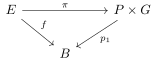We have a $$G{\hbox{-}}$$isomorphism $$E_1 \xrightarrow{f} E_2$$, and so a composition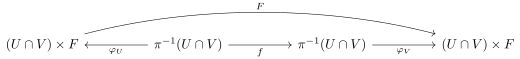Here we’ve used that $$f$$ commutes with the projection maps. We want to show $$\operatorname{im}(F) \subseteq G$$. We have a compositeWe can fill this in to a commutative diagram: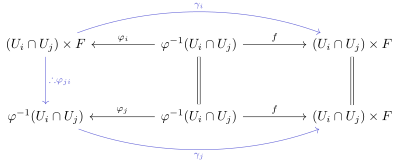The converse direction proceeds similarly!

A $$G{\hbox{-}}$$isomorphism between the bundles $$E_1, E_2$$ obtained from clutching data $$\left\{{\phi_{ij}}\right\}$$ and $$\left\{{\psi_{ij}}\right\}$$ respectively with the same cover $$\left\{{U_i}\right\}_{i\in I}$$ give maps $$\gamma_i: U_i\to G$$ such that \begin{align*} \gamma_j \phi_{ji} \gamma_i^{-1}= \psi_{ji} .\end{align*}

We can form the composite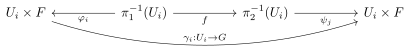And then assemble a commuting diagram:## 5.1 Nonabelian Čech Cohomology

Let $${\mathcal{U}}\mathrel{\vcenter{:}}=\left\{{U_i}\right\}_{i\in I}\rightrightarrows B$$ an open cover, and define \begin{align*} {\check{C}}^0({\mathcal{U}}; G) \mathrel{\vcenter{:}}=\left\{{ \left\{{\gamma_i: U_i\to G}\right\}_{i\in I} }\right\} ,\end{align*} which is a group under pointwise multiplication. Define \begin{align*} {\check{C}}^2({\mathcal{U}}; G) &\mathrel{\vcenter{:}}=\left\{{ \left\{{\phi_{ij}: U_i \cap U_j \to G}\right\}_{i, j\in I} }\right\}\\ {\check{C}}^3({\mathcal{U}}; G) &\mathrel{\vcenter{:}}=\left\{{ \left\{{\phi_{ijk}: U_i \cap U_j \cap U_k \to G}\right\}_{i,j,k \in I} }\right\} ,\end{align*} and boundary maps \begin{align*} \delta^{0}: {\check{C}}^0({\mathcal{U}}; G) &\to {\check{C}}^1({\mathcal{U}}; G)\\ \left\{{\gamma_i: U_i\to G}\right\} &\mapsto \left\{{\phi_{ji} \mathrel{\vcenter{:}}=\gamma_j\gamma_i^{-1}: U_i \cap U_j \to G }\right\} ,\end{align*}

\begin{align*} \delta^{1}: {\check{C}}^1({\mathcal{U}}; G) &\to {\check{C}}^2({\mathcal{U}}; G)\\ \left\{{\phi_{ij}: U_i \cap U_j \to G}\right\} &\mapsto \left\{{\eta{ijk} \mathrel{\vcenter{:}}=\phi_{ij} \phi_{jk} \phi_{ik}^{-1}: U_i \cap U_j \cap U_k \to G }\right\} .\end{align*}

One can check that $$\delta^1 \circ \delta^0 = 0$$ is trivial. And 1-cocycle will yield a fiber bundle.

A bundle is trivial iff it is a 1-coboundary, where we take $$Z^1({\mathcal{U}}; G) \mathrel{\vcenter{:}}=\ker \delta^1$$, $$B^1({\mathcal{U}}; G) \mathrel{\vcenter{:}}=\operatorname{im}\delta^0$$.

We’d like to define homology as $$Z/B$$, but since these aren’t abelian groups, the coboundaries $$B$$ may not be normal in $$Z$$ and the quotient may not yield a group.

There is an action of $${\check{C}}^0({\mathcal{U}}; G)\curvearrowright{\check{C}}^1({\mathcal{U}}; G)$$ given by taking $$\gamma \mathrel{\vcenter{:}}=\left\{{\gamma_i}\right\}_{i\in I}$$ and setting $$(\gamma \phi)_{ij} = \gamma_i \phi_{ij} \gamma_j^{-1}$$, which descends to an action on $$Z^1$$. We can take the quotient by this action to define \begin{align*} {\check{H}}^1({\mathcal{U}}; G) \mathrel{\vcenter{:}}= Z^1({\mathcal{U}}; G) / \sim .\end{align*}

Two bundles are isomorphic iff they yield the same element in $${\check{H}}^1({\mathcal{U}}; G)$$.

This works when bundles have the same open cover, and if not, we can take a common refinement.

# 6 Tuesday, September 07

Recall that given a $$B\in {\mathsf{Top}}$$ and $${\mathcal{U}}\rightrightarrows B$$, we defined $${\check{H}}_1({\mathcal{U}}; G)$$ which classified isomorphism classes of fiber bundles $$E \xrightarrow{\pi} B$$ with fiber $$F$$, $$G \subseteq {\operatorname{Homeo}}(F)$$, and structure group $$G$$, given by clutching data using $${\mathcal{U}}$$. The cochains were given by the following:

\begin{align*} {\check{C}}^0({\mathcal{U}}; G) &= \left\{{\left\{{ \gamma_i: U_i \to G}\right\}_{i\in I}}\right\}\\ {\check{C}}^1({\mathcal{U}}; G) &= \left\{{\left\{{ \phi_{ij}: U_i \cap U_j \to G }\right\}_{i, j \in I}}\right\}\\ {\check{C}}^2({\mathcal{U}}; G) &= \left\{{\left\{{ \eta_{ijk}: U_i \cap U_j \cap U_k \to G }\right\}_{i,j,k\in I}}\right\} \end{align*} with boundary maps $$\delta_i: {\check{C}}^{i-1} \to {\check{C}}^i$$: \begin{align*} (\delta_1 \gamma)_{ij} &= \gamma_i \gamma_j^{-1}\\ (\delta_2 \phi)_{ijk} &= \phi_{ij} \phi_{jk} \phi_{ik}^{-1} .\end{align*} Note that

• $$\delta_2 \circ \delta_1 = 0$$
• $$\ker \delta_2 = Z^1({\mathcal{U}}; G)$$ yields clutching data, i.e. a fiber bundle with fiber $$F$$,
• $$\operatorname{im}\delta_1$$ yields trivial bundles,
• $${\check{H}}^1({\mathcal{U}}; G) \mathrel{\vcenter{:}}= Z^1({\mathcal{U}}; G) / \operatorname{im}({\check{C}}^0({\mathcal{U}}; G) \to Z^1({\mathcal{U}}; G))$$.

We’ll see that $$(\gamma \varphi)_{ij} = \gamma_i \varphi_{ij} \gamma_j^{-1}$$, and by a lemma this will prove the above claim about classifying isomorphism classes.

We say a cover $${\mathcal{V}}\mathrel{\vcenter{:}}=\left\{{V_j}\right\}_{j\in J}$$ is a refinement of $${\mathcal{U}}\mathrel{\vcenter{:}}=\left\{{U_i}\right\}_{i\in I}$$ iff there exists a function $$f:J\to I$$ between the index sets where $$V_j \subseteq U_{f(j)}$$ for all $$j$$.

DZG: I’ll write $${\mathcal{V}}\leq {\mathcal{U}}$$ if $${\mathcal{V}}$$ is a refinement of $${\mathcal{U}}$$.

Since any two covers have a common refinement, we’ll assume $${\mathcal{V}}\leq {\mathcal{U}}$$ is always a refinement. We can then restrict clutching data from $${\mathcal{U}}$$ to $${\mathcal{V}}$$: given $$\left\{{\phi_{ij}}\right\}_{i,j\in I}$$, we can set $$\psi_{ij} \mathrel{\vcenter{:}}={ \left.{{ \phi_{f(i), f(j)}}} \right|_{{V_i \cap V_j}} }$$, noting that if $$V_j \subseteq U_{f(j)}$$ and $$V_i \subseteq U_{f(i)}$$ then $$V_i \cap V_j \subseteq U_{f(i)} \cap U_{f(j)}$$. These yield maps $$\psi_{ij}: V_i \cap V_j \to G$$ satisfying the cocycle condition, so $$\psi_{ij} \in Z^1({\mathcal{V}}; G)$$. This means that we have map $$Z^1({\mathcal{U}}; G)\to Z^1({\mathcal{V}}; G)$$ which respects the actions of $${\check{C}}^0({\mathcal{U}}; G), {\check{C}}^0({\mathcal{V}}; G)$$ respectively. Since the category of covers with morphisms given by refinements come from a preorder, we can take a colimit to define \begin{align*} {\check{H}}^1(B; G) \mathrel{\vcenter{:}}=\colim_{{\mathcal{U}}\rightrightarrows B} {\check{H}}^1({\mathcal{U}}; G) .\end{align*}

There is a bijection \begin{align*} \left\{{\substack{ \text{Fiber bundles with fiber }F \\ \text{and structure group }G }}\right\}_{/ {\sim}} &\rightleftharpoons {\check{H}}^1(B; G) \end{align*} In particular, these classes are independent of $$F$$.

There is an equivalence of categories \begin{align*} \left\{{\substack{ \text{Fiber bundles with fiber $F$} \\ \text{and structure group }G \\ \text{over }B }}\right\}_{/ {\sim}} &\rightleftharpoons \mathop{\mathrm{Prin}}{\mathsf{Bun}}(G) _{/ {B}} ,\end{align*} where the right-hand side are principal $$G{\hbox{-}}$$bundles.

Given a map $$G \to {\operatorname{Homeo}}(F)$$, a $$G{\hbox{-}}$$structure on an $$F{\hbox{-}}$$bundle $$E \xrightarrow{\pi} B$$ is the following data: given clutching data $$\phi_{ij}$$, lifts of the following form that again satisfy the cocycle condition:Note that we need to impose the cocycle condition, since lifts may not be unique and some choices may not glue correctly!

Using the known $${\operatorname{Spin}}$$ double covers, we can form the composition \begin{align*} {\operatorname{Spin}}_n({\mathbb{R}}) \xrightarrow{\times 2} {\operatorname{SO}}_n({\mathbb{R}}) \hookrightarrow{\operatorname{Homeo}}({\mathbb{R}}^n) .\end{align*} Then a $${\operatorname{Spin}}_n{\hbox{-}}$$structure on any $${\mathbb{R}}^n{\hbox{-}}$$bundle is a lift of transition functions from $${\operatorname{Homeo}}({\mathbb{R}}^n)$$ to $${\operatorname{Spin}}_n$$ satisfying the cocycle condition.

We can fill in a commutative square in the following way: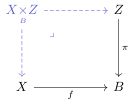Here we can construct the fiber product as \begin{align*} X{ \underset{\scriptscriptstyle {B} }{\times} } Z = \left\{{(x, e) {~\mathrel{\Big\vert}~}\pi(e) = f(x)}\right\} .\end{align*}

It satisfies the following universal property: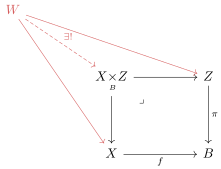If $$\pi: P\to X$$ is a principal $$G{\hbox{-}}$$bundle and $$f:Y\to X$$ is a continuous map, then the following highlighted portion of the pullback is again a principal $$G{\hbox{-}}$$bundle: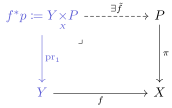We in fact obtain $${\operatorname{pr}}_1^{-1}(y) = \pi^{-1}(f(y))\cong G$$, and there will be a right $$G{\hbox{-}}$$action on each fiber. Behold this gnarly diagram:If $$P\to X$$ is trivial, this says the pullback will be trivial and $$U\times G\mapsto f^{-1}(U)\times G$$ will be a homeomorphism.

So the functor $$X\mapsto \mathop{\mathrm{Prin}}_G(X)$$ is contravariant functor.

Suppose $$B$$ is paracompact and Hausdorff, then there is a principal $$G{\hbox{-}}$$bundle $$P \xrightarrow{\pi} I \times B$$. Consider the fiber bundle $$P_0 \mathrel{\vcenter{:}}={ \left.{{P}} \right|_{{\left\{{0}\right\}\times B}} } \to B$$, then there is a diagram:This extends to an isomorphism $$I\times P_0 \to I\times B$$ and $$P\to I\times B$$:$$P_1 = { \left.{{P}} \right|_{{1\times B}} } \cong P_0$$.

If $$f_0 \sim f_1: Y\to X$$ are homotopic and $$P\to X$$, then $$f_0^*P \cong f_1^* P$$.

Use the homotopy lifting property to get a map $$h$$:Then $${ \left.{{h^* P}} \right|_{{0\times Y}} } \simeq{ \left.{{h^*P}} \right|_{{1\times Y}} } \cong f_1^*P$$.

# 7 Thursday, September 09

## 7.1 Corollaries of the homotopy bundle lemma

Last time: the bundle homotopy lemma. If $$P\to I\times X \in \mathop{\mathrm{Prin}}{\mathsf{Bun}}(G)$$, then there is a bundle isomorphism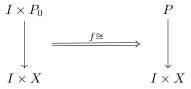where $${ \left.{{f}} \right|_{{0\times P_0}} }$$ is the identity.
If $$P\to I\times X \in \mathop{\mathrm{Prin}}{\mathsf{Bun}}(G)$$ then $$P_0 \cong P_1$$ where $$P_i \mathrel{\vcenter{:}}={ \left.{{P}} \right|_{{i\times X}} }$$.
If $$f_0, f_1: Y\to X$$ with $$P \xrightarrow{\pi} X$$, then $$f_0^* P \cong f_1^* P$$ are isomorphic bundles.
If $$X$$ is contractible, then any $$P\in \mathop{\mathrm{Prin}}{\mathsf{Bun}}(G)_{/ {X}}$$ is trivial.